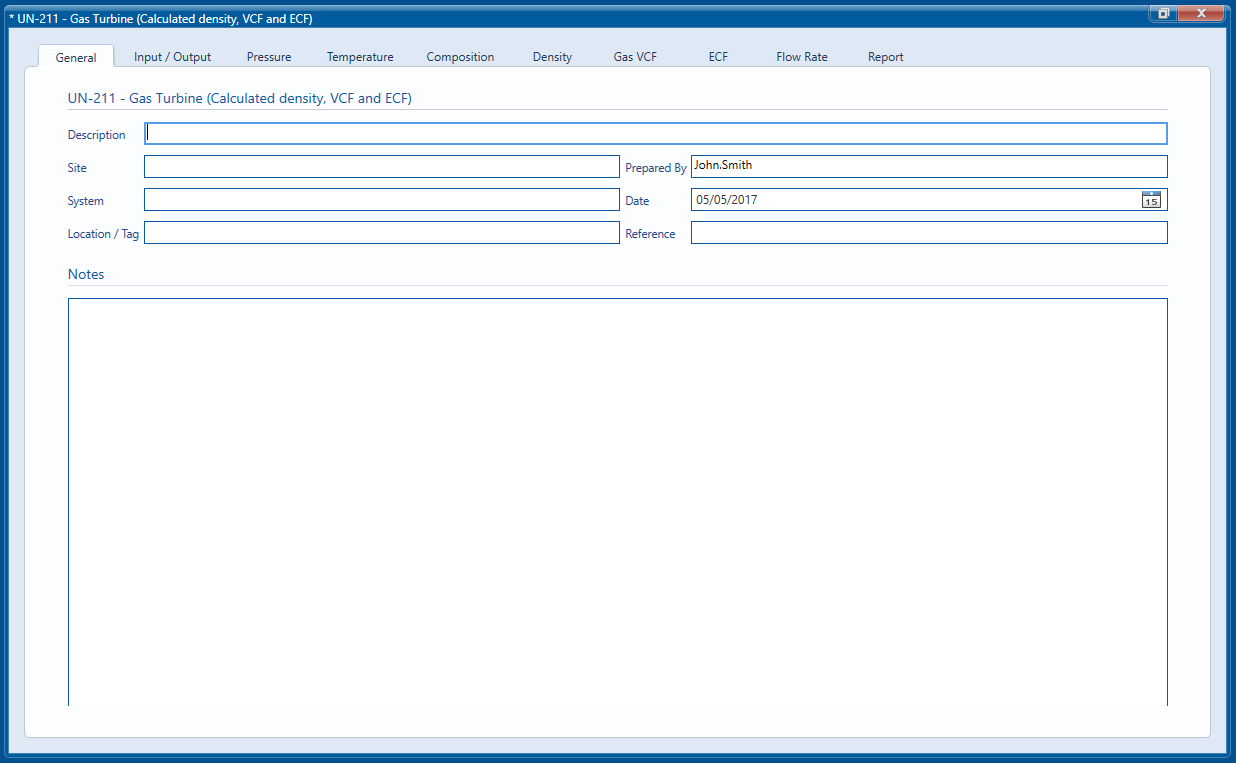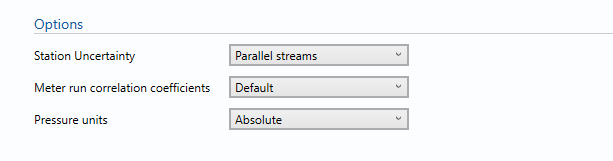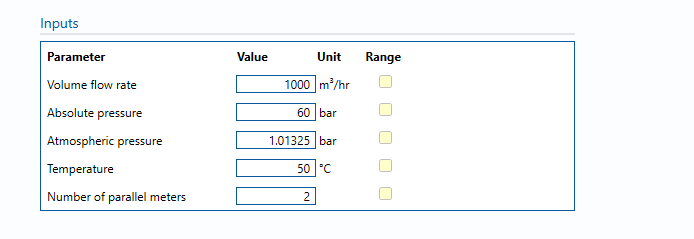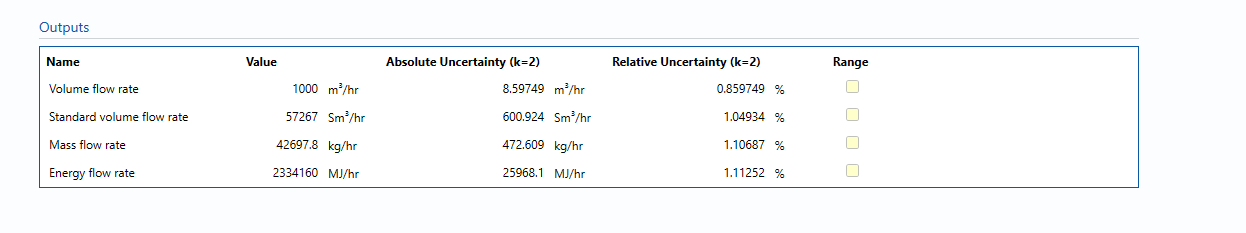# UN-211: Gas Turbine (Calculated Density, VCF and ECF)

## Description

Calculates the uncertainty in observed volume, mass, standard volume and energy flowrate for a gas turbine meter. Meter flowrate uncertainties are determined from an assessment of the meter calibration performance, installation and on-going performance/usage parameters. Density, standard density and CV calculations and their uncertainties are performed using user-selectable calculations. Pressure and temperature uncertainties are determined using the technical specification for the transmitters selected from the integrated transmitter selection tool.

## General

The General tab is used to enter information, which is saved along with the uncertainty calculation and included in reports which are generated.The tabs used for inputting data and calculating the uncertainty in the individual components of the uncertainty can be seen along the top of the module window.

The report tab is only visible once the calculation has been run and will not be visible if any parameters are changed until the calculation has been run once more.

## Input/output

### OptionsThe options section controls how the calculation is performed and dictates which inputs are required.

#### Station Uncertainty

By default, the module will calculate the uncertainty for a single metering stream. If parallel streams are selected the Station Uncertainty will be calculated assuming the metering streams are nominally identical and the flow is split equally between the streams in use.

Note: When selecting parallel streams the correlation between inputs has to be considered and the user is presented with a further option to control how the correlation is defined.

#### Pressure Units

This is the line pressure of the gas stream measured upstream of the Coriolis meter.

Note: As described above, if Absolute is selected inputs are required for Absolute and Atmospheric pressure and if Gauge is selected inputs are required for Gauge and Atmospheric pressure.

The default value for Absolute Pressure is set to 1 standard atmosphere (1.01325 bar)

#### Meter run correlation coefficients

• Default
• User entered

usually a number that is between 0 and 1 that shows the relationship between the different streams.

### Inputs#### Volume flow rate

The volume flow rate of the gas passing through the gas turbine meter.

#### Pressure

This is the line pressure of the gas stream measured. As described above, if Absolute is selected inputs are required for Absolute and Atmospheric pressure and if Gauge is selected inputs are required for Gauge and Atmospheric pressure.

#### Temperature

This is the measured temperature of the gas stream.

#### Number of parallel meters

This input is only visible when parallel streams are selected for the station uncertainty and refers to the number of in-use metering streams the flow is divided between.

### OutputsThe outputs section shows the calculated flow rate and uncertainty taken from the Flow Rate tab.

### Calculation Blocks

The following blocks are used within this uncertainty module:

Pressure

Temperature

Composition

Density

Gas VCF

ECF

Flow Rate

Back